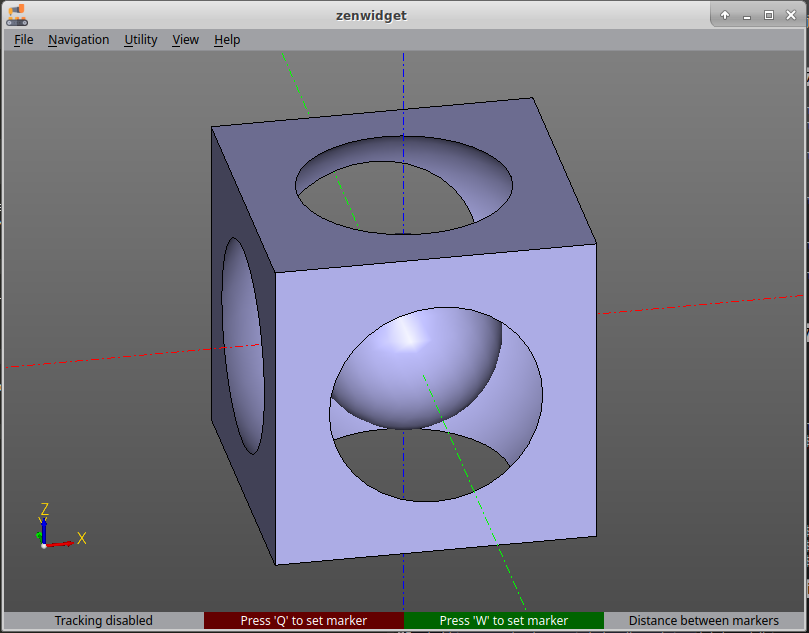Hello Friend.

Here is an example to demonstrate the principle of building models in zencad.

a = box(200, 200, 200, center = True)
b = sphere(120)
c = sphere(60)

model = a - b + c

display(model)

show()

What's happening:

In the first line, we import into the current zencad namespace. In this case, we are interested in the box,sphere, display,show functions.

a = box(200, 200, 200, center = True)
b = sphere(120)
c = sphere(60)

Preparing geometric primitives. A box object is created with dimensions 200x200x200 and an offset of the geometric center to the origin. It also creates two spheres with a radius of 120 and 60.

model = a - b + c

Computing the model using boolean operations. First, a large sphere will be subtracted from the cube. Then a small one was added. The order of the terms is important in this case, since the operations of union and difference of geometric bodies are non-commutative.

disp(model)

The disp function passes the object into the scene for later display.

show()

Displaying the scene widget.

If everything went well: